## research papersJOURNAL OFSYNCHROTRONRADIATION
ISSN: 1600-5775
Volume 6| Part 5| September 1999| Pages 1031-1034

## A synchrotron radiation X-ray scattering study of aqueous solutions of native DNA

aDipartimento di Chimica Inorganica, Università di Palermo, I-90128 Palermo, Italy, and bEuropean Molecular Biology Laboratory, Outstation Hamburg, EMBL, c/o DESY, D-22603 Hamburg, Germany
*Correspondence e-mail: koch@embl-hamburg.de

(Received 25 April 1999; accepted 4 June 1999)

Synchrotron radiation small-angle X-ray scattering (SAXS) was used to investigate solutions of native DNA at different ionic strengths and temperatures. The mass per unit length, radius of gyration of the cross-section of DNA and apparent second virial coefficient (A2) were obtained from Zimm plots in the rodlike particle approximation. The values of A2 obtained in this way are positive and almost constant indicating that the repulsive interactions still influence the scattering patterns at resolutions as high as 5–8 nm. SAXS measurements in continuous temperature scans indicate that the rod approximation is valid over a wide temperature range during DNA melting and confirm that the rodlike–wormlike transition temperature increases with ionic strength.

### 1. Introduction

The high charge per unit length of DNA, due to the negatively charged phosphate groups along the backbone of the double helix, strongly influences its behaviour in aqueous solution. The intramolecular electrostatic repulsion makes polynucleotides very rigid and the resulting large persistence length allows them to be modelled as thin rods of infinite length. Using this property, Manning (1978) and Record et al. (1978) developed a theoretical framework to interpret the non-specific interactions between ions and biopolymers in solution. Some aspects of these theories have recently been re-examined (Stigter, 1995; Duguid & Bloomfield, 1996), and the properties of DNA solutions as functions of added salt concentration and temperature have been investigated by small-angle neutron scattering and dynamic light scattering (Borsari et al., 1998). Small-angle X-ray scattering has been previously used to establish the rodlike behaviour of DNA in solution (Luzzati et al., 1967) and study the short-range order due to electrostatic repulsion (Koch et al., 1995) at low ionic strength as well as the thermal denaturation of DNA (Puigdomenech et al., 1989). The influence of the ionic strength on the conformation of stiff and flexible polyelectrolytes, and the problem of polyelectrolyte expansion in low salt solutions, have been extensively studied by light scattering (Borochov & Eisenberg, 1994). Studies on DNA (Borochov et al., 1981) are of special interest because, despite the fact that DNA is the paradigm of polyelectrolytes with a high charge/length ratio, little is known about the balance of repulsive and attractive forces (Ise & Matsuoka, 1994) that determine its solution structure and properties. In the present work the effect of ionic strength and of the temperature-induced DNA denaturation on the scattering patterns were further investigated.

### 2. Materials and methods

Calf thymus or E. coli DNA (Sigma or Serva Feinbiochemica) were re-suspended in 1 mM tris-HCl, pH 7.5, and dialysed, for at least 6 h, against 1 mM tris-HCl, 5 mM edta, pH 7.5, and five changes of 1 mM tris-HCl. The dialysis bag (Visking, MW cut-off 12000–14000 Da) was previously treated following standard procedures (McPhie, 1971).

All DNA solutions and buffers were extensively degassed under vacuum prior to the measurements. The X-ray scattering experiments were performed on the double-focusing monochromator mirror camera X33 (Koch & Bordas, 1983) of the EMBL in HASYLAB on the storage ring DORIS III of the Deutsches Electronen Synchrotron (DESY) using a linear or quadrant position-sensitive gas detector with delay line readout (Gabriel & Dauvergne, 1982) for room- and variable-temperature experiments, respectively, with the standard data acquisition and evaluation systems (Boulin et al., 1986, 1988). The observation range, using a 2 m camera, was 0.01 ≤ s ≤ 0.4 nm−1, where s = 2sinθ/λ, 2θ is the scattering angle and λ = 0.15 nm is the wavelength of the incident radiation. The scattering patterns of DNA solutions (1.77–5.63 mg ml−1) in 1 mM tris-HCl and between 0 and 150 mM NaCl were recorded in 1 min time frames at room temperature as well as during temperature scans between 303 and 373 K, at a rate of 2 K min−1. The solvent scattering was subtracted, the data were normalized to the intensity of the primary beam and corrected for the detector response following standard procedures implemented in the program SAPOKO (D. I. Svergun & M. H. J. Koch, unpublished). The radius of gyration of the cross-section, Rc, and the forward scattering, Ic(0), corresponding to the relative mass per unit length (ML) were calculated in a range where interference effects were negligible and the relation 4π2s2Rc2 ≤ 1 is valid (Feigin & Svergun, 1987), using the programs OTOKO (Boulin et al., 1986) and GNOM (Svergun et al., 1988; Svergun, 1992). The mass per unit length of DNA was established by reference to the forward scattering of a freshly prepared solution (8.73 mg ml−1) of bovine serum albumin (BSA) (Sigma) in 50 mM hepes, pH 7.5, measured under identical conditions, assuming a molecular weight of 66.5 kDa for BSA.

DNA concentrations of dilute samples were measured on a Kontron Uvikon 922 spectrophotometer taking = 7000 cm−1M−1 at λ = 258 nm (Kennedy & Bryant, 1986) and assuming a molecular weight of 315 Da per mononucleotide, and the relative concentrations were verified by comparing the X-ray scattering intensities of the DNA solutions in the range s > 0.15 nm−1, where interparticle interference was negligible (see §3).

### 3. Results and discussion

#### 3.1. Room temperature

The values of Rc, 1.1 ± 0.02 nm, and ML, 1.7 ± 0.1 kDa nm−1, calculated from the slope and the intercept of log[sI(s)] versus s2 plots, are independent on DNA concentration, biological source and ionic strength, within experimental error, as previously observed (Koch et al., 1995). This also implies that there is no significant separation into single strands as occasionally reported in the literature (Borochov & Eisenberg, 1994), even at the lowest ionic strengths used here. Zimm plots (Zimm, 1948; Flory & Bueche, 1958) in the rodlike approximation {[sI(s)]−1 versus (100s2 + c)}, for different DNA concentrations and five ionic strength conditions (Fig. 1), should be more sensitive to intermolecular interactions than individual cross-section plots. Linear fits were made in the ranges 0.1 ≤ s ≤ 0.22 nm−1 for 0 mM NaCl and 0.07 ≤ s ≤ 0.15 nm−1 for higher ionic strengths. This procedure, which assumes rodlike linear chains, gives the values of Rc from the slope of the line for c = 0 and of ML from the average of the intercepts at c = 0 and s = 0. These values are independent of the length distribution of native DNA (Feigin & Svergun, 1987). The values of ML for DNA (Fig. 1and Table 1) are in agreement with the theoretical value of 1850 Da nm−1, calculated using 315 Da as the average molecular weight per mononucleotide (50% G–C composition) and taking 0.34 nm as the axial distance between monomer units in B-DNA (Arnott & Hukins, 1972), and with that obtained by light scattering (2100 Da nm−1) (Kirste & Oberthuer, 1982).

 Table 1Mass per unit length, ML, radius of gyration of the cross-section, Rc, and apparent second virial coefficient, A2, of native calf thymus DNA in 1 mM tris-HCl, pH = 7.5, solutions at different NaCl concentrations, determined from the Zimm plots in the rodlike approximation (Fig 1)
[NaCl] (mM) ML (Da nm−1) Rc (nm) A2 (1013 cm4 mg−1 Da−1)
0 1775 ± 140 1.22 ± 0.10 1.8 ± 3.4
10 1781 ± 70 1.17 ± 0.17 6.6 ± 3.6
50 1711 ± 20 1.06 ± 0.09 6.2 ± 2.7
100 1643 ± 50 1.03 ± 0.16 3.5 ± 2.5
150 1739 ± 70 1.15 ± 0.14 7.6 ± 4.7
†The values of the mass per unit length of DNA, ML, were calculated by the relation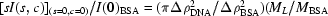) (see e.g. Feigin & Svergun, 1987), where Δρ2 is the contrast of the scattering particles in water, MBSA, 66500 Da, is the molecular weight of BSA, I(0)BSA, 34.95 ± 0.15 (relative units), is the forward-scattering of BSA, found in the globular particles approximation, using the same experimental conditions; the values of sI(s,c) (in relative units) at s = 0 and c = 0 were calculated as the average of the intercepts, at s = 0 and c = 0, of the Zimm plot (see Fig. 1).
‡The values of Rc and A2 were found from the Zimm equations (Zimm, 1948; Kirste & Oberthuer, 1982) modified for rodlike polymers, where K is a constant depending on the experimental conditions: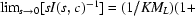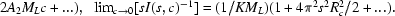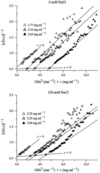Figure 1 Zimm plots of calf thymus DNA solutions at different concentrations, as indicated, in 1 mM tris-HCl and 0 mM or 150 mM NaCl.

The values of the apparent second virial coefficient were obtained from the slope of the s = 0 line. They are constant and positive within experimental error indicating that the effects of repulsive interactions, although much weaker than at lower scattering angles, can still be detected at resolutions as high as 5–8 nm. Zimm plots rely on the assumption that the single-particle scattering function does not depend on concentration. Plots of A2 against ionic strength, however, rely on the further – usually implicit – assumption that the single-particle scattering function is also independent of ionic strength. This is verified in the rodlike scattering region (s = 0.1–0.2 nm−1) but may not be the case in the light-scattering region (s < 0.01 nm−1) (Borochov & Eisenberg, 1994) as the persistence length and hence single-particle scattering strongly depends on ionic strength between 0 and 150 mM NaCl (Puigdomenech et al., 1989).

This in turn should affect the apparent dependence of A2 on ionic strength and may also result in a dependence of A2 measured by light scattering on the contour length.

#### 3.2. Variable temperature

Fig. 2displays two scattering patterns of E. coli DNA at different temperatures and NaCl concentrations. Similar results were obtained with calf thymus DNA. The linear trend of the log[sI(s)] versus s2 plots for s > 0.1 nm, at all ionic strengths, indicates that the rod approximation is also valid at high temperatures. The interference maximum around s = 0.05 nm−1 at very low ionic strength (see, for example, Fig. 2a, 0 mM NaCl) persists at high temperatures, indicating that the short-range order of DNA solutions under low ionic strength conditions (Koch et al., 1995) still exists in solution of thermally denatured DNA. The decrease in the slope and intercept of the linear part of the plots with temperature (i.e. the decrease of the radius of gyration of the cross-section and of the mass per unit length) follows the thermal denaturation of DNA, from double to single strands conformation, as previously reported (Puigdomenech et al., 1989).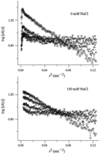Figure 2 Log [sI(s)] versus s2 plots at various temperatures for 5 mg ml−1 E. coli DNA in 1 mM tris-HCl solutions and 0 mM or 150 mM NaCl. T = 303 K (○), 343 K (⋄), 363 K (▵), 373 K (▿).

The integrated intensities in two different s-ranges are plotted in Fig. 3as correlation plots (Koch et al., 1987; Koch, 1991) for different NaCl concentrations. In all cases there is a linear trend over a wide temperature range (303–351 K in 1 mM tris-HCl, 303–373 K in the presence of 150 mM NaCl), where the hypothesis of the presence of double- and single-stranded DNA particles during melting is valid. At very low ionic strength (0 mM NaCl) the change of slope in the correlation plot above 353 K reflects a change in the melting mechanism. At higher ionic strength this break occurs at higher temperatures, and is no longer observed in the presence of 150 mM NaCl (see Fig. 3). This change in slope of the correlation plot at high temperature and low ionic strength in all likelihood corresponds to the end of the double to single strand transition of DNA and the onset of the rodlike–wormlike transition. Beyond this breakpoint the SAXS can no longer be interpreted in the rodlike particle approximation. As already noted by others (Borochov & Eisenberg, 1994), single-stranded polyribonucleotides chains are intrinsically less stable than double-stranded ones and undergo transitions which are no longer reversible. Note that the slope of the correlation becomes steeper above 10 mM NaCl suggesting that there is also a slight change in the DNA structure.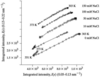Figure 3 Correlation plots of the integrated intensity data in the range 0.13 ≤ s ≤ 0.22 nm−1 and 0.05 ≤ s ≤ 0.13 nm−1 at the same temperature for solutions of E. coli DNA 5 mg ml−1 in tris-HCl 1 mM and different NaCl concentrations as indicated on the right-hand side of each curve. Successive curves have been displaced by 500 along the ordinate for better visualization. Both axes shown in arbitrary units.

### 4. Conclusions

Synchrotron radiation small-angle X-ray scattering was used to study aqueous solutions of native DNA containing between 0 and 150 mM NaCl in the temperature range 303 ≤ T ≤ 373 K. Previous X-ray and neutron studies on DNA in solution (Koch et al., 1995; Borsari et al., 1998) gave clear evidence for strong repulsive interactions leading to interparticle interference, in the absence of added salt. This long-range electrostatic repulsion among the negatively charged DNA rods vanishes in the presence of screening counterions, in physiological conditions (150 mM NaCl). The use of Zimm plots in the rodlike approximation yields values of the apparent second virial coefficient which correspond to an almost ideal behaviour with slightly repulsive interactions.

This suggests that the strong repulsive interactions leading to a rapid increase of the second virial coefficient observed in light scattering and to short-range order in the region 100–10 nm still significantly affect the scattering pattern at resolutions around 5–8 nm even in dilute solutions. A fortiori, these effects should be taken into account when studying more concentrated systems.

Two types of transitions are clearly detected in the correlation plots of the SAXS data at variable temperature: the thermal denaturation, involving the separation of double strands into single strands, and the rodlike–wormlike transition, which involves coiling and irreversible denaturation. The latter transition is no longer detectable in the temperature range of the observations for NaCl concentrations above 10 mM. The slight increase in slope of the correlation plots above 10 mM NaCl suggests that a subtle change in the structure of DNA in solution may occur around this value of ionic strength.

### Footnotes

Present address: Sabançi University, Faculty of Engineering and Natural Sciences, 80020 Istanbul, Turkey.

### Acknowledgements

GB thanks the EMBL for fellowships and support under the European Union (HCMP Access to Large Installations Programme, contract No. CHGE-CT93-0040).

### ReferencesJOURNAL OFSYNCHROTRONRADIATION